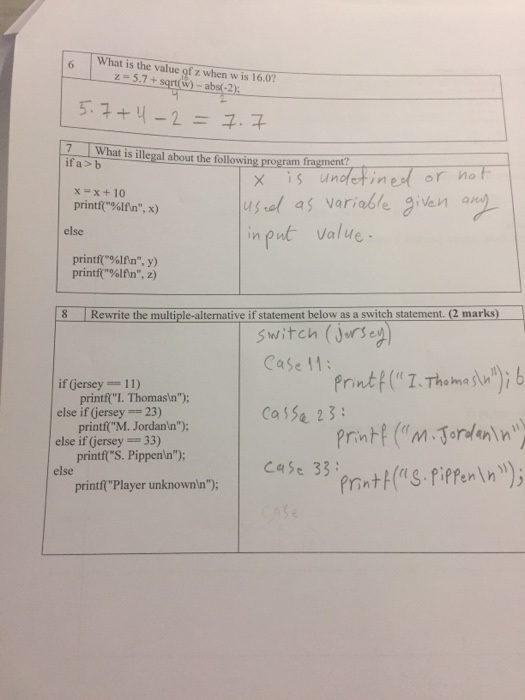# Question & Answer: What is the value of z when w is 16.0? z = 5.7 + squareroot (w) – abs(-2): What is illegal about the following program fragment? if a > b…..What is the value of z when w is 16.0? z = 5.7 + squareroot (w) – abs(-2): What is illegal about the following program fragment? if a > b x = x = 10 printf(“%1fn”, x) else printf(“%1fn”, y) printf(“%lfn”, z) Rewrite the multiple-alternative if statement below as a switch statement. if(jersey == 11) printf(“I. Thomasn”): else if (jersey == 23) printf(“M. Jordann”) else if(jersey ==33) printf(“S. Pippenn”): else printf(“Player unknownn”);

Don't use plagiarized sources. Get Your Custom Essay on
Question & Answer: What is the value of z when w is 16.0? z = 5.7 + squareroot (w) – abs(-2): What is illegal about the following program fragment? if a > b…..
GET AN ESSAY WRITTEN FOR YOU FROM AS LOW AS \$13/PAGE

The value of z when w is 16:

=> z = 5.7 + sqrt(w) – abs(-2)

=> z = 5.7 + sqrt(16) – abs(-2) : Put the value of w in square root function

=> z = 5.7 + 4 – 2 : because square root of 16 is 4

: absolute value of abs(-2) is 2

=> z = 9.7 – 2 : First we have to execute the (+) operator because + is higher execution priority then (-)operator and we have to execute the expression from Left to right.

abs() function: The abs() function returns the absolute value of a number. The absolute value of a number is the number without its sign. (For reference only)

Ques => What is illegal about the following program fragment:

=> The following things are illegal according to c programming standard in the given program fragment:

1) if -else condition is not opened { and closed } by bracket.

2) Semicolon (;) has not used at the end of statements:

3) In if statement a>b condition not written in bracket.

Correct Program Fragment is:

if (a>b) {

x = x+10;

printf(“%1fn”, x);

}

else {

printf(“%1fn”, y);

printf(“%1fn”, z);

}

Rewrite the multiple-alternative if statement below as switch statement:

int jersey; /* local variable definition */ /*Just for understanding */
//Enter the value of jersey through input function /*Just for understanding */

switch(jersey) {
case 11 :
printf(“I. Thomasn” );
break;
case 23 :
printf(“M. Jordann” );
break;
case 33 :
printf(“S. Pippenn” );
break;
default :
printf(“Player unknownn” );
}